# 非对称性Anderson晶格模型中的磁性相变研究Magnetic Transition in Non-Symmetric Anderson Lattice Model

• 全文下载: PDF(1183KB)    PP.23-31   DOI: 10.12677/CMP.2019.81004
• 下载量: 386  浏览量: 1,361   国家自然科学基金支持

Anderson晶格模型是描述重费米子系统的重要理论模型，而隶玻色子方法是求解该模型的常用方法。然而，通常的Kotliar-Ruckenstein隶玻色子方法只能处理该模型的粒子–空穴对称情形。本文将Kotliar-Ruckenstein方法加以推广，使之能够处理偏离粒子–空穴对称的非对称情形，并用之研究了非对称性Anderson晶格模型中的磁性相变，展示了晶格维度、电子跳迁系数、库仑关联强度、电子杂化强度、局域电子能级等多种参数对磁性相变边界的影响。

Anderson lattice model, which can be solved by the widely used slave-boson method, is a standard model to describe the heavy-fermion systems. However, the normal Kotliar-Ruckenstein slave-boson method is limited to the case with particle-hole symmetry, particular in investigating the magnetic phase in Anderson lattice model. In this paper, the Kotliar-Ruckenstein method is generalized to include the non-symmetric case which violates the particle-hole symmetry. Then, the magnetic phase boundary in Anderson lattice model is studied as a function of lattice dimension, electron-hopping amplitudes, Coulomb correlation, electron hybridization and local energy level.

1. 引言

2. 模型与计算方法

$\begin{array}{c}H=\underset{i,j,\sigma }{\sum }\left({t}_{ij}^{d}{d}_{i\sigma }^{†}{d}_{j\sigma }+{t}_{ij}^{f}{f}_{i\sigma }^{†}{f}_{j\sigma }\right)+{ϵ}_{f}\underset{i,\sigma }{\sum }\text{\hspace{0.17em}}{f}_{i\sigma }^{†}{f}_{i\sigma }\\ \text{\hspace{0.17em}}\text{\hspace{0.17em}}+U\underset{i}{\sum }\text{\hspace{0.17em}}{n}_{i↑}^{f}{n}_{i↓}^{f}\text{+}V\underset{i,\sigma }{\sum }\left({d}_{i\sigma }^{†}{f}_{\sigma }+h.c.\right)\\ \text{\hspace{0.17em}}\text{\hspace{0.17em}}-\mu \underset{i,\sigma }{\sum }\left({d}_{i\sigma }^{†}{d}_{i\sigma }+{f}_{i\sigma }^{†}{f}_{i\sigma }\right)\end{array}$ (1)

$\begin{array}{l}H=\underset{i}{\sum }\left(U{\delta }_{i}+{h}_{i}{m}_{i}^{f}-{\eta }_{i}{n}_{i}^{f}\right)+\underset{i,j,\sigma }{\sum }\left({t}_{ij}^{d}-\mu {\delta }_{ij}\right){d}_{i\sigma }^{†}{d}_{j\sigma }+\underset{i,\sigma }{\sum }\left({ϵ}_{f}+{\eta }_{i}-\sigma {h}_{i}-\mu \right){f}_{i\sigma }^{†}{f}_{i\sigma }\\ \text{\hspace{0.17em}}\text{\hspace{0.17em}}\text{\hspace{0.17em}}\text{\hspace{0.17em}}\text{\hspace{0.17em}}+\underset{i,j,\sigma }{\sum }\text{\hspace{0.17em}}{t}_{ij}^{f}{Z}_{i\sigma }{Z}_{j\sigma }{f}_{i\sigma }^{†}{f}_{j\sigma }+V\underset{i,\sigma }{\sum }\left({Z}_{i\sigma }{d}_{i\sigma }^{†}{f}_{i\sigma }+h.c.\right)\end{array}$ (2)

$\begin{array}{l}{Z}_{1}=\frac{\sqrt{2}\left(\sqrt{\left({n}_{f}-2\delta +{m}_{f}\right)\left(1-{n}_{f}+\delta \right)}+\sqrt{\delta \left({n}_{f}-2\delta -{m}_{f}\right)}\right)}{\sqrt{\left({n}_{f}+{m}_{f}\right)\left(2-{n}_{f}-{m}_{f}\right)}},\\ {Z}_{2}=\frac{\sqrt{2}\left(\sqrt{\left({n}_{f}-2\delta -{m}_{f}\right)\left(1-{n}_{f}+\delta \right)}+\sqrt{\delta \left({n}_{f}-2\delta +{m}_{f}\right)}\right)}{\sqrt{\left({n}_{f}-{m}_{f}\right)\left(2-{n}_{f}+{m}_{f}\right)}}\end{array}$ (3)

$H=N\left(U\delta +h{m}_{f}-\eta {n}_{f}\right)+\underset{k\in \text{MBZ}}{\sum }\text{\hspace{0.17em}}{\Psi }_{k}^{†}{H}_{k}{\Psi }_{k},$ (4)

${\Psi }_{k}={\left({d}_{kA↑},{d}_{kA↓},{d}_{kB↑},{d}_{kB↓},{f}_{kA↑},{f}_{kA↓},{f}_{kB↑},{f}_{kB↓}\right)}^{\text{T}}$ ，哈密顿矩阵为

${H}_{k}=\left(\begin{array}{cc}{H}_{k}^{d}& {V}_{k}\\ {V}_{k}^{+}& {H}_{k}^{f}\end{array}\right),$ (5)

${V}_{k}=V\left(\begin{array}{cccc}{Z}_{1}& 0& 0& 0\\ 0& {Z}_{2}& 0& 0\\ 0& 0& {Z}_{2}& 0\\ 0& 0& 0& {Z}_{1}\end{array}\right),$

${H}_{k}^{f}=\left(\begin{array}{cccc}{e}_{1k}& 0& {Z}_{1}{Z}_{2}{t}_{f}{\lambda }_{k}& 0\\ 0& {e}_{2k}& 0& {Z}_{1}{Z}_{2}{t}_{f}{\lambda }_{k}\\ {Z}_{1}{Z}_{2}{t}_{f}{\lambda }_{k}& 0& {e}_{2k}& 0\\ 0& {Z}_{1}{Z}_{2}{t}_{f}{\lambda }_{k}& 0& {e}_{1k}\end{array}\right),$

3. 粒子–空穴对称情况

$H=N\left(U\delta +h{m}_{f}-U/2\right)+\underset{k\in \text{MBZ,}\sigma }{\sum }\text{\hspace{0.17em}}{\Psi }_{k\sigma }^{†}{H}_{k\sigma }{\Psi }_{k\sigma },$ (6)

$Q=\left(\pi ,\pi ,\pi \right)$ (三维)或 $Q=\left(\pi ,\pi \right)$ (二维)，哈密顿量矩阵为

${H}_{k\sigma }=\left(\begin{array}{cccc}{\epsilon }_{k}& 0& VZ& 0\\ 0& -{\epsilon }_{k}& 0& VZ\\ VZ& 0& \alpha {Z}^{2}{\epsilon }_{k}& 0\\ 0& VZ& 0& -\alpha {Z}^{2}{\epsilon }_{k}\end{array}\right),$ (7)

$\begin{array}{l}{E}_{k}^{±}=\frac{1}{\sqrt{2}}\sqrt{{E}_{1k}±\sqrt{{E}_{1k}^{2}-{E}_{2k}^{2}}},\\ {E}_{1k}=\left(\text{1+}{\alpha }^{2}{Z}^{4}\right){\epsilon }_{k}^{2}+{h}^{2}+2{V}^{2}{Z}^{2},\\ {E}_{2k}=\sqrt{4{\epsilon }_{k}^{2}{h}^{2}+4{Z}^{4}{\left({V}^{2}-\alpha {\epsilon }_{k}^{2}\right)}^{2}}\end{array}$ (8)

$\begin{array}{l}U-\frac{1}{N}\underset{k\in \text{BZ}}{\sum }\frac{2Z}{\sqrt{{E}_{1k}+{E}_{2k}}}\left[{V}^{2}+{t}_{f}^{2}{Z}^{2}{\epsilon }_{k}^{2}+\frac{2{Z}^{2}{\left({V}^{2}-{t}_{f}{\epsilon }_{k}^{2}\right)}^{2}}{{E}_{2k}}\right]\cdot \frac{\partial Z}{\partial \delta }=0,\\ {m}_{f}-\frac{1}{N}\underset{k\in \text{BZ}}{\sum }\frac{h}{\sqrt{{E}_{1k}+{E}_{2k}}}\left(1+\frac{2{\epsilon }_{k}^{2}}{{E}_{2k}}\right)=0,\\ h=U\cdot \frac{\partial Z}{\partial {m}_{f}}/\frac{\partial Z}{\partial \delta }\end{array}$ (9)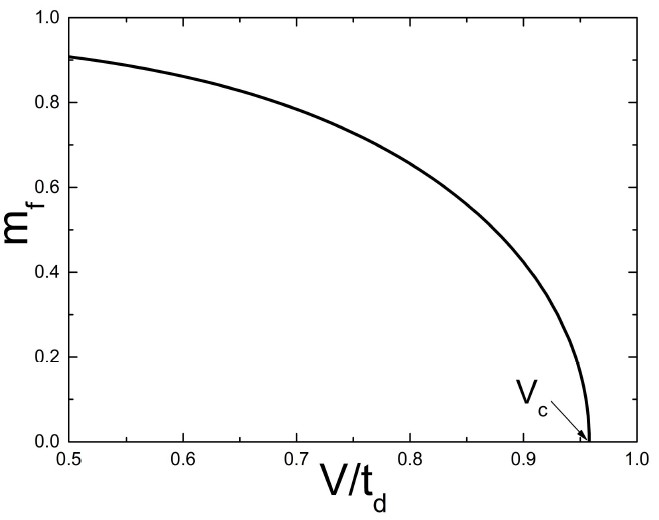Figure 1. The staggered magnetization ${m}_{f}$ of the symmetric Anderson lattice model vs d-f hybridization V in the cubic lattice. At critical ${V}_{c}$ , an antiferromagnetic transition takes place. Model parameters: ${t}_{f}=-0.2$ , $U=4$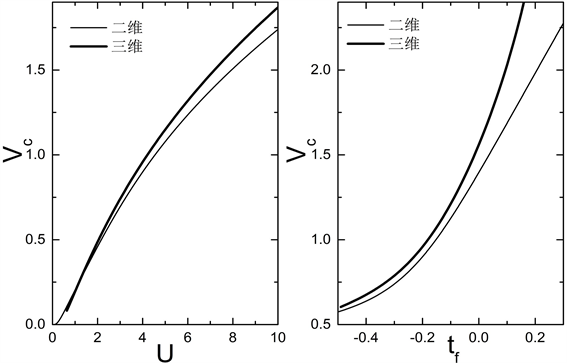Figure 2. Antiferromagnetic transition point of the symmetric Anderson lattice model vs Coulomb correlation energy U (left) or ${t}_{f}$ (right) in square (thin lines) and cubic lattice (thick lines). Parameters: ${t}_{f}=-0.2$ for left and $U=4$ for right

4. 非粒子–空穴对称情况

${E}_{g}=N\left(U\delta +h{m}_{f}-\eta {n}_{f}+\mu {n}_{t}\right)+\underset{k\in \text{MBZ}}{\sum }{\left({H}_{k}\right)}_{nm}{〈nm〉}_{k},$ (10)

$U+\frac{1}{N}\underset{n,m,k\in \text{MBZ}}{\sum }\frac{\partial {\left({H}_{k}\right)}_{nm}}{\partial \delta }{〈nm〉}_{k}=0,$ (11)

$\begin{array}{c}{〈nm〉}_{k}\equiv 〈{\left({\Psi }_{k}^{†}\right)}_{n}{\left({\Psi }_{k}\right)}_{m}〉\\ =〈\underset{i}{\sum }{\left({\Phi }_{k}^{†}\right)}_{i}{\left({U}_{k}\right)}_{ni}^{\ast }\underset{j}{\sum }{\left({U}_{k}\right)}_{mj}{\left({\Phi }_{k}\right)}_{j}〉\\ =\underset{i=1}{\overset{8}{\sum }}{\left({U}_{k}\right)}_{ni}^{\ast }{\left({U}_{k}\right)}_{mi}\Theta \left(-{E}_{k}^{\left(i\right)}\right)\end{array}$ (12)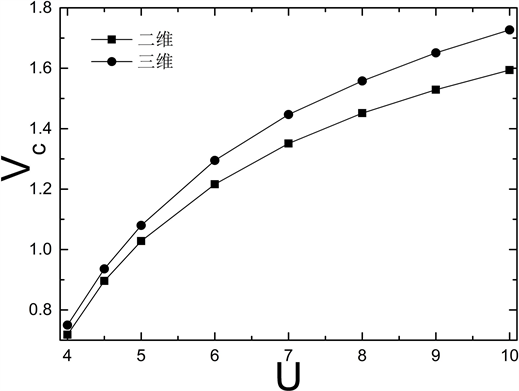Figure 3. The antiferromagnetic transition point ${V}_{c}$ of the non-symmetric Anderson lattice model vs Coulomb correlation energy U in the square (square dots) and cubic lattice (circle dots). Parameters: ${\epsilon }_{f}=-3$ , ${t}_{d}=0.15$ , ${t}_{f}=-0.2$ , ${{t}^{\prime }}_{f}=-0.02$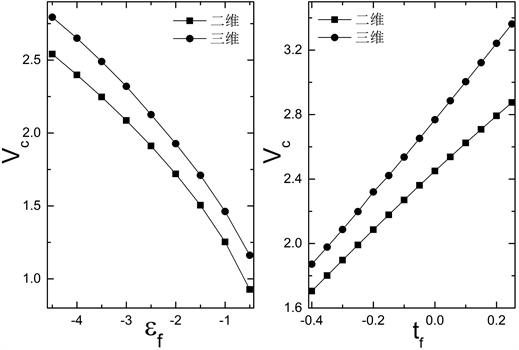Figure 4. The antiferromagnetic transition point ${V}_{c}$ of the non-symmetric Anderson lattice model in large U limit vs f level ${\epsilon }_{f}$ (left) or ${t}_{f}$ (right) in the square lattice (square dots) and cubic lattice (circle dots). Parameters: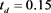, ${t}_{f}=-0.2$ , ${{t}^{\prime }}_{f}=-0.02$ for left; ${\epsilon }_{f}=-3$ , ${t}_{d}=0.15$ , ${{t}^{\prime }}_{f}=0.1{t}_{f}$ for right

5. 结论

  Andres, K., Graebner, J.E. and Ott, H.R. (1975) 4f-Virtual-Bound-State Formation in CeAl3 at Low Temperatures. Physical Review Letters, 35, 1779. https://doi.org/10.1103/PhysRevLett.35.1779  Hewson, A.C. (1997) The Kondo Problem to Heavy Fermions. Cambridge University Press, Cambridge.  Li, H., Liu, Y., Zhang, G.-M. and Yu, L. (2015) Phase Evolution of the Two-Dimensional Kondo Lattice Model near Half-Filling. Journal of Physics: Condensed Matter, 27, Article ID: 425601. https://doi.org/10.1088/0953-8984/27/42/425601  Nemkovski, K.S., Mignot, J.M., Alekseev, P.A., Ivanov, A.S., Nefeodova, E.V., Rybina, A.V., Regnault, L.P., Iga, F. and Takabatake, T. (2007) Polarized-Neutron Study of Spin Dynamics in the Kondo Insulator YbB12. Physical Review Letters, 99, Article ID: 137204. https://doi.org/10.1103/PhysRevLett.99.137204  Liu, Y., Li, H., Zhang, G.-M. and Yu, L. (2012) d-Wave Superconductivity Induced by Short-Range Antiferromagnetic Correlations in the Two-Dimensional Kondo Lattice Model. Physical Review B, 86, Article ID: 024526. https://doi.org/10.1103/PhysRevB.86.024526  Xu, N., Biswas, P.K., Dil, J.H., Dhaka, R.S., Landolt, G., Muff, S., Matt, C.E., Shi, X., Plumb, N.C., Radovic, M., Pomjakushina, E., Conder, K., Amato, A., Borisenko, S.V., Yu, R., Weng, H.-M., Fang, Z., Dai, X., Mesot, J., Ding, H. and Shi, M. (2014) Article | Published: 30 July 2014 Direct Observation of the Spin Texture in SmB6 as Evidence of the Topological Kondo Insulator. Nature Communications, 5, Article Number: 4566. https://doi.org/10.1038/ncomms5566  Schnyder, A.P. and Ryu, S. (2011) Topological Phases and Surface Flat Bands in Superconductors without Inversion Symmetry. Physical Review B, 84, Article ID: 060504(R). https://doi.org/10.1103/PhysRevB.84.060504  Laia, H.-H., Grefea, S.E., Paschenc, S. and Si, Q.M. (2018) Weyl-Kondo Semimetal in Heavy-Fermion Systems. Proceedings of the National Academy of Sciences, 115, 93-97. https://doi.org/10.1073/pnas.1715851115  李正中. 固体理论[M]. 第二版. 北京: 高等教育出版社, 2002.  Bauer, E., Hilscher, G., Michor, H., Paul, Ch., Scheidt, E.W., Gribanov, A., Seropegin, Y., Noël, H., Sigrist, M. and Rogl, P. (2004) Heavy Fermion Superconductivity and Magnetic Order in Noncentrosymmetric CePt3Si. Physical Review Letters, 92, Article ID: 027003. https://doi.org/10.1103/PhysRevLett.92.027003  Vekic, M., Cannon, J.W., Scalapino, D.J., Scalettar, R.T. and Sugar, R.L. (1995) Competition between Antiferromagnetic Order and Spin-Liquid Behavior in the Two-Dimensional Periodic Anderson Model at Half Filling. Physical Review Letters, 74, 2367. https://doi.org/10.1103/PhysRevLett.74.2367  Sun, S.-J., Hong, T.-M. and Yang, M.F. (1995) Phase Diagram of the Two-Dimensional Periodic Anderson Model at Half-Filling. Physica B: Condensed Matter, 216, 111. https://doi.org/10.1016/0921-4526(95)00429-7  Li, H., Zhong, Y., Liu, Y., Luo, H.-G. and Song, H.-F. (2018) z2 Classification for a Novel Antiferromagnetic Topological Insulating Phase in Three-Dimensional Topological Kondo Insulator. Journal of Physics: Condensed Matter, 30, Article ID: 435601. https://doi.org/10.1088/1361-648X/aae17b